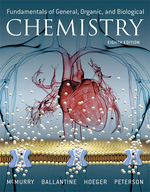×
Get Full Access to Fundamentals Of General, Organic, And Biological Chemistry (Mastering Chemistry) - 8 Edition - Chapter 7.3 - Problem 7.5
Get Full Access to Fundamentals Of General, Organic, And Biological Chemistry (Mastering Chemistry) - 8 Edition - Chapter 7.3 - Problem 7.5

×ISBN: 9780134015187 2044

## Solution for problem 7.5 Chapter 7.3

Fundamentals of General, Organic, and Biological Chemistry (Mastering Chemistry) | 8th Edition

• Textbook Solutions
• 2901 Step-by-step solutions solved by professors and subject experts
• Get 24/7 help from StudySoup virtual teaching assistantsFundamentals of General, Organic, and Biological Chemistry (Mastering Chemistry) | 8th Edition

4 5 1 260 Reviews
23
4
Problem 7.5

How much heat is absorbed (in kilojoules) during production of 127 g of NO by the combination of nitrogen and oxygen?

$$\mathrm{N}_{2}(g)+\mathrm{O}_{2}(g) \longrightarrow 2 \mathrm{NO}(g) \quad \Delta H=+180 \mathrm{~kJ} / \mathrm{mol}$$

Text Transcription:

N_2(g)+ O_2(g) longrightarrow 2 NO (g) quad/Delta H=+180~kJ/mol

Step-by-Step Solution:
Step 1 of 3

Rachel Ferrell CHEM 1030 3/21-­‐3/24 Chapter 7: Molecular Geometry: • Valence Shell Electron Pair Repulsion Model (VSEPR) Model o AB x o A= central atom o X= can be any integer 2-­‐6 • Basic idea of model→electrons repel each other, therefore they will arrange themselves to be as far apart as possible from each other • Creates Electron Domains, which can be… o Lone pairs o Single bonds o Double bonds o Triple bonds • Electron domain geometry= arrangement of electron domains around a central atom o Assuming that the electron domains are all bonds and not lone pairs… o 2 electron domains→ linear o 3 electron domains→ trigonal planar o 4 electron domains→ tetrahedral o 5 electron domains→ trigonal bipyramidal o 6 electron domains→ octahedral • Molecular geometry= arrangement of bonded atoms o Is not always the same as electron domain geometry o Electron domain geometry is used t

Step 2 of 3

Step 3 of 3

##### ISBN: 9780134015187

Unlock Textbook Solution